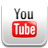# Ohms Law Formula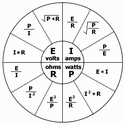By Dave Rongey Summary: Ohms Law Formula and how it is used for determining electrical loads as it pertains to Watts, Volts and Amps. Ohms Law is very helpful to help us understand sizing electrical circuits for the home electrical system.

## Basic Ohms Law Formula for Electrical Wiring Calculations

The Ohm's Law Calculations for Electrical

Using the Ohm's Law is a way to determine information needed to understand how to design electrical circuits.

This formula works with three main variables and depending on what is known can produce a missing factor which is needed to determine the circuit size.

The Ohm's Law is also used when designing appliances and building electrical equipment and devices.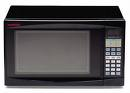Example: A Microwave Oven circuit Circuit Planning: Device ________________ Volts _________________ Amps ________________ Quantity ______________ Circuit Size Amps ______ Wire Size ____________ See the Wire Size Chart Ohms Law Formula to Convert Watts: (calculating watts to amps) Watts / Volts x Quantity = *Total Amps. * Note: This does not include Power Factor or Voltage Drop (wire distance). Knowing the specified circuit size as amps will not require the Ohms Law Calculation.

## Understanding Electrical Equipment and Appliance Labels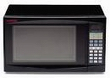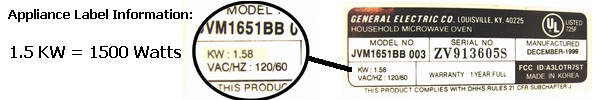## Electrical Calculations and Formulas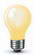### Converting Watts to Kilowatt/hours

Question:
Can you tell me how to convert watts to kilowatt/hours?

Kilowatts represents 1000 watts, so 6000 watts is the same as 6 kw.

Based on this, if 6000 watts of power were consumed for one hour then that would be 6000 watts per hour.

A watt hour meter measures how many watts of power are consumed per hour. Electrical utility bills often include the peak demand period, which is the highest amount of electricity that is consumed in one hour. If this high demand of electricity is during the peak demand period of the day then this may have an effect on the electrical utility bill causing it to be more expensive. If a high amount of electricity is consumed during non-peak demand periods during the day then the electrical billing may not be as costly.
Be sure to get your copy of my BIG New eBook:

Complete Guide to Home Electrical Wiring

Wire It Right with the help of my Illustrated Wiring Book
Great for any Home Wiring Project.

## See How to Wire it Right!

Perfect for Homeowners, Students and Electricians
Includes:
Home Electrical Wiring - Room by Room
120 Volt Circuits
240 Volt Circuits
Multi-Wired Circuits
Wiring Methods for Installing Home Electrical Circuit Wiring
Electrical Codes for Home Electrical Wiring
....and much more.